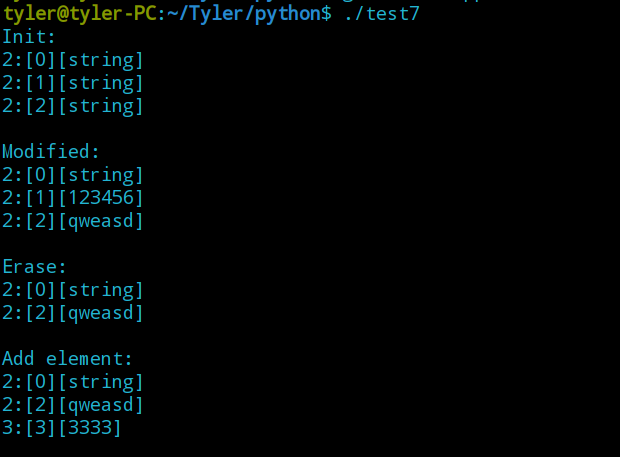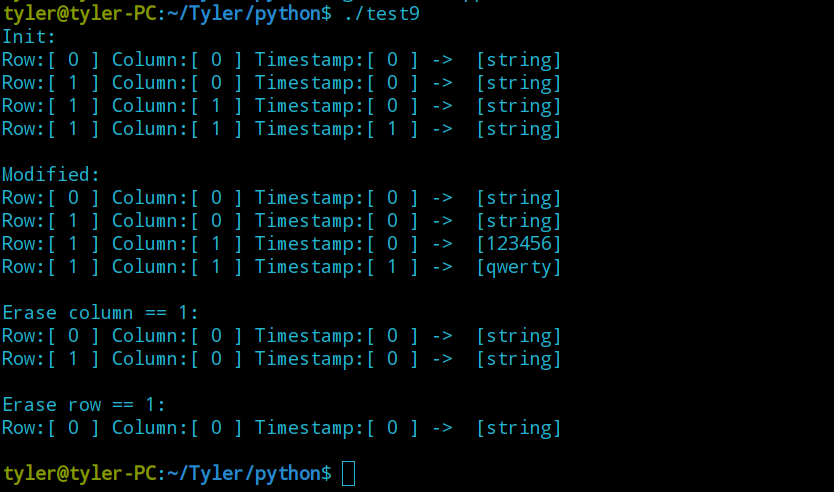## 二维 map

``map<string, map<string, string>> b;``

(2) 增加单独的数据可以使用赋值方法 test[ "维度1" ][ "维度2" ] = " 字符串 "；，这种方法既简单又方便。若原容器中没有相应的 key ，则完成插入操作，反之完成赋值操作。

``````for(int i = 0; i < 3; i++)
{
temp = to_string(i);
c.insert(make_pair(temp, "string"));
}

//将含有3个元素的 map c 插入到 map b中
b.insert(make_pair(temp, c));

//添加一个元素
b["2"]["1"] = "123456";
``````

``````b["2"].erase("1");

b.erase("1");

``````void Print(map<string, map<string, string>> a)
{
map<string, map<string, string>>::iterator p2;
map<string, string>::iterator p3;

for(p2 = a.begin(); p2 != a.end(); p2++)
{
for(p3 = p2->second.begin(); p3 != p2->second.end(); p3++)
cout << p2->first << ":" << "[" <<p3->first << "]" << "[" << p3->second << "]" <<endl;
}
cout <<endl;
}``````

``````#include <map>
#include <iostream>
int main()
{
std::map<int, std::string> c = {
{1, "one"}, {2, "two"}, {3, "three"},
{4, "four"}, {5, "five"}, {6, "six"}};

for(auto it = c.begin(); it != c.end(); )
if(it->first % 2 == 1)
//完成相应操作
else
++it;
for(auto& p : c)
std::cout << p.second << ' ';
}``````

``````#include <iostream>
#include <map>
#include <string>

using namespace std;

void Print(map<string, map<string, string>> a)
{
map<string, map<string, string>>::iterator p2;
map<string, string>::iterator p3;

for(p2 = a.begin(); p2 != a.end(); p2++)
{
for(p3 = p2->second.begin(); p3 != p2->second.end(); p3++)
cout << p2->first << ":" << "[" <<p3->first << "]" << "[" << p3->second << "]" <<endl;
}
cout <<endl;
}

int main()
{
map<string, map<string, string>> b;
map<string, string> c;
string temp;

//对 map c 进行初始化
for(int i = 0; i < 3; i++)
{
temp = to_string(i);
c.insert(make_pair(temp, "string"));
}

//将 map c 插入到 map b 中
b.insert(make_pair(temp, c));
cout << "Init:" <<endl;
Print(b);

//修改数据
b["2"]["1"] = "123456";
b["2"]["2"] = "qweasd";
cout << "Modified:" <<endl;
Print(b);

b["2"].erase("1");
cout << "Erase:" <<endl;
Print(b);

b["3"]["3"] = "3333";
Print(b);

return 0;
}``````## 三维 map

``````#include <iostream>
#include <map>
#include <string>
#include <vector>

using namespace std;

void Print(map<string, map<string, map<string, string>>> a)
{
map<string, map<string, map<string, string>>>::iterator p1;
map<string, map<string, string>>::iterator p2;
map<string, string>::iterator p3;
for(p1 = a.begin(); p1 != a.end(); p1++)
{
for(p2 = p1->second.begin(); p2 != p1->second.end(); p2++)
{
for(p3 = p2->second.begin(); p3 != p2->second.end(); p3++)
cout << "Row:[ " << p1->first << " ] Column:[ " << p2->first << " ] Timestamp:" << "[ " <<p3->first << " ]" << " ->  [" << p3->second << "]" <<endl;
}
}
cout <<endl;
}

int main()
{
map<string, map<string, map<string, string>>> a;
map<string, map<string, string>> b;
map<string, string> c;
string temp;

//初始化并插入
for(int i = 0; i < 2; i++)
{
temp = to_string(i);
c.insert(make_pair(temp, "string"));
b.insert(make_pair(temp, c));
a.insert(make_pair(temp, b));
}
cout << "Init:" <<endl;
Print(a);

//修改
a["1"]["1"]["0"] = "123456";
a["1"]["1"]["1"] = "qwerty";
cout << "Modified:" <<endl;
Print(a);

a["1"].erase("1");
cout << "Erase column == 1:" <<endl;
Print(a);

a.erase("1");
cout << "Erase row == 1:" <<endl;
Print(a);

return 0;
}``````### pythonwhile输出每一个余数_Python学习（5）（while循环语句、循环嵌套、break/continue、赋值运算符、转义字符等 ）..._weixin_39946274的博客-程序员宝宝

Python学习(5)一、python的while 循环语句二、python的赋值运算符三、python的while 循环语句 示例四、python的 break 和 continue五、python的while 嵌套1、用嵌套打印小星星2、python 九九乘法表六、python的print 函数的补充(不自动换行)七、python的字符串中的转义字符程序的三大流程顺序----从上向下，顺序执行...

### 【AndroidStudio】 “错误: 程序包R不存在“，����: �����R������，乱码R乱码 的解决方法_zZnamesystem的博客-程序员宝宝_程序包r不存在

【AndroidStudio】 "错误: 程序包R不存在"，����: �����R������，乱码R乱码 的解决方法